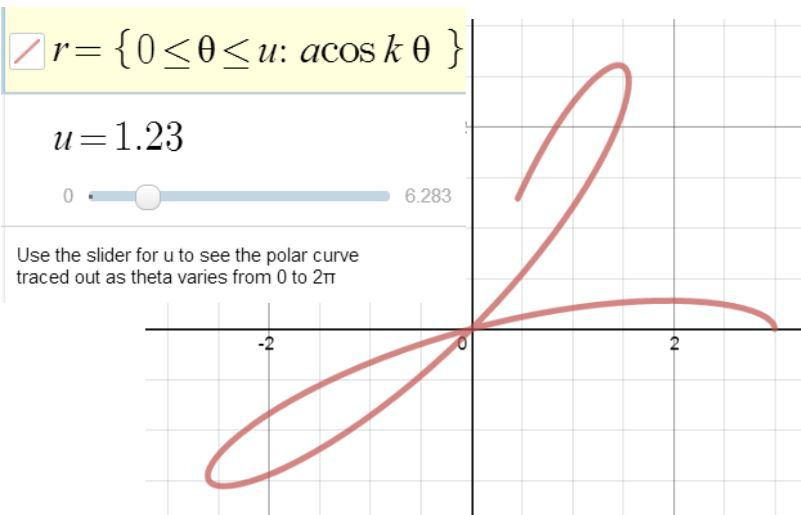# Polar Coordinates – ResourcesWith Year 13 I will be looking at Polar Coordinates this week. The first thing we’ll need to do is understand the meaning of polar coordinates and be able to convert from polar to Cartesian coordinates and vice-versa. So we will need some polar graph paper and Desmos! Checking my stationary list I found exactly what I needed on MathBits, scroll down the page for polar papers; one of the options usefully provides 4 smaller grids to one page.

So now to plot some points, Desmos provides a good solution

With two points to play with, we can understand how negative values of r and θ are displayed and appreciate that a point may be described in more than one way with polar coordinates.

The Desmos page also shows the relationship between polar and Cartesian coordinates (the Cartesian form is needed to plot the points.) On that subject, Desmos created the page below – match up the two points.We also need to be able to plot polar curves, Desmos is ideal for exploring polar curves; it is possible to use sliders to see how the curve is generated as values of θ increase.On the AQA website the Teaching and learning resources page for A Level Further Maths includes three online textbooks under the Resources for students heading. The second book (Unit 03) includes a chapter on Polar Coordinates.

WolframAlpha can also be used for polar plots.

For further examples and resources, see this post on Mathematics for students.